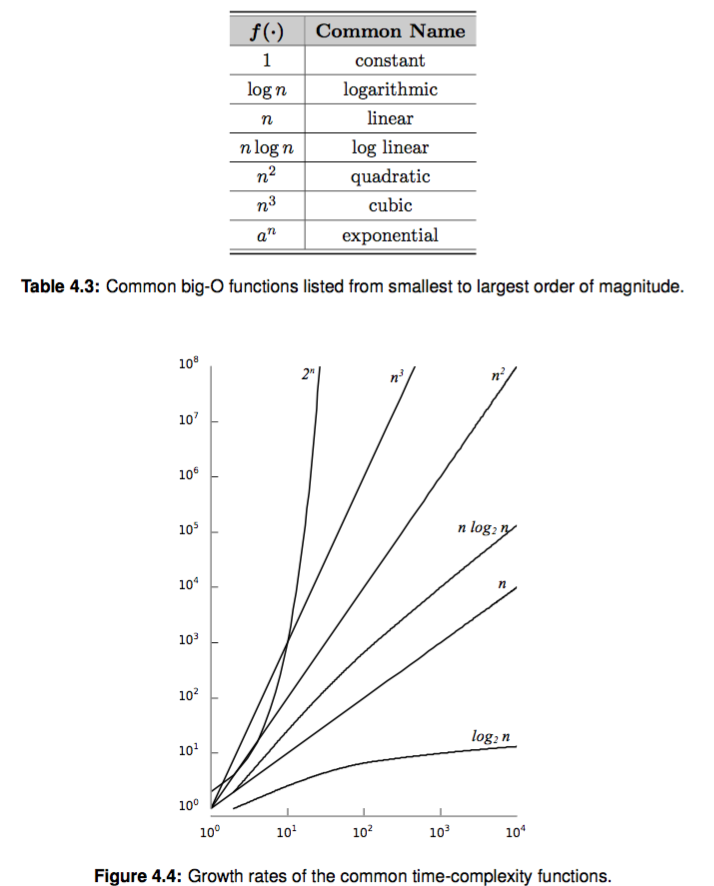# 大 O 表示法

# version1
total_sum = 0
for i in range(n):
row_sum[i] = 0
for j in range(n):
row_sum[i] = row_sum[i] + matrix[i, j]
total_sum = total_sum + matrix[i, j]

# version2
total_sum = 0
for i in range(n):
row_sum[i] = 0
for j in range(n):
row_sum[i] = row_sum[i] + matrix[i, j]
total_sum = total_sum + row_sum[i]    # 注意这里和上边的不同


v1 版本的关键操作在 j 循环里，两步加法操作，由于嵌套在第一个循环里，操作步骤是 $(2n) * n = 2n^2$

v2 版本的 total_sum 只有 n 次操作，它的操作次数是 $n + n*n = n^2 + n$

n $2n^2$ $n^2 +n$
10 200 110
100 20,000 10,100
1000 2,000,000 1,001,000
10000 200,000,000 100,010,000
100000 20,000,000,000 10,000,100,000

# 如何计算时间复杂度

• v1: $2n*n = 2n^2$
• v2: $n + n*n = n + n^2$

• v1: $2n*n = 2n^2$
• v2: $n + n*n = n + n^2 \leq 2n^2$

# 常用时间复杂度

O 名称 举例
1 常量时间 一次赋值
$\log n$ 对数时间 折半查找
$n$ 线性时间 线性查找
n$\log n$ 对数线性时间 快速排序
$n^2$ 平方 两重循环
$n^3$ 立方 三重循环
$2^n$ 指数 递归求斐波那契数列
$n!$ 阶乘 旅行商问题

# 常见复杂度增长趋势图# 思考题

• 回头看看前几章我们讲到的数据结构，以及每个操作的时间复杂度，你能理解了吗？
• 二分查找是针对有序元素的一种经典的查找算法，你知道的它的时间复杂度吗？你能简单证明下吗。
• 斐波那契数列你肯定很熟悉，它的公式是 F(n) = F(n-1) + F(n-2)，你知道计算一个斐波那契数 F(n) 的时间复杂度吗？你会用数学公式证明吗？
• 你能指出时间和空间权衡的例子吗？往往很多高效的数据结构能同时兼顾时间和空间复杂度，但是有时候我们却得做出一定的权衡

# 参考资料

(本章我用了 MathJax 来书写一些简单的数学公式，使用 "\$"包含起来的就是数学公式)